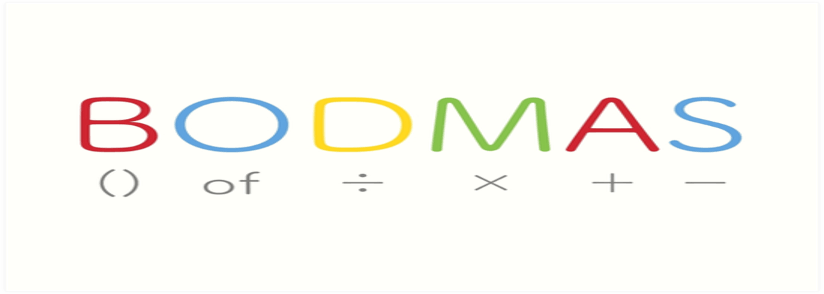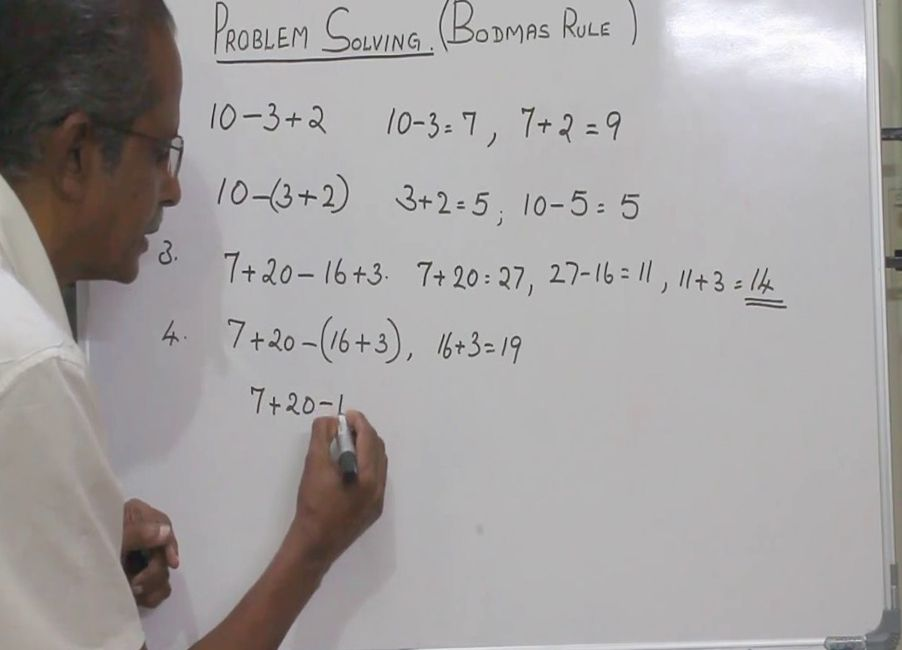# What is a BODMAS Rule and How It Is Applied in Mathematics

TeachingKids and parents often have basic queries about BODMAS, so the first question is, what is BODMAS in math? BODMAS in math, or the BODMAS rule, outlines the sequence of operations to adhere to while performing calculations. Students must grasp the correct order of operations to arrive at accurate answers, especially when working with a BODMAS worksheet. This guide aims to assist you in comprehending how to apply this rule to various math problems.

## What is BODMAS?

What does BODMAS stand for? BODMAS stands for Brackets, Orders (powers and roots), Division, Multiplication, Addition, and Subtraction. The interpretation of the rule is the following: you have to solve brackets first, then orders (powers, square roots), division and multiplication, and lastly addition and subtraction.

## What is the BODMAS rule?

The BODMAS math rules state that calculations should be carried out in the following sequence: first, solve expressions within brackets; then, handle orders involving powers and square roots; next, perform division and multiplication operations; and finally, execute addition and subtraction. Understanding and applying this rule correctly is fundamental to solving math problems accurately.

Here is how this works:

### Numbers

Numbers are the objects that allow us to count things, compare amounts, and make calculations. We do not give numbers priority in BODMAS. We treat them as equals, whether they are small or large.

Math for Kids

Is Your Child Struggling With Math?
1:1 Online Math Tutoring### Operators

Operators are symbols that perform mathematical operations on a value. They are applicable in expressions, which are groups of symbols that evaluate a single value. Also, mathematical operators are symbols that represent an action, such as addition or multiplication. Operators do specific actions when used in mathematical equations. These are:

## BODMAS examples

The order of operations is always important. For example, let’s take the expression.

3 + 2 x 5 =

Since there are no brackets and orders, you must perform multiplication before you add the numbers. This is because multiplication has a higher precedence than addition. You can check BODMAS worksheets for more examples to teach kids. And if you want to set assessments, you can use BODMAS examples with answers.

## BODMAS Meaning

BODMAS acronym, which stands for Brackets, Orders, Division, Multiplication, Addition, and Subtraction, outlines the specific sequence of operations to be followed when calculating multiple steps. Adhering to this order is crucial, as deviating from it can lead to incorrect answers.## How to use BODMAS Rule

These are the steps on how to use the BODMAS rules.

• If there are brackets, solve them first
• If there are no brackets, solve orders (exponents and roots) next.
• If there are no orders, perform multiplication and division.
• If there are no multiplication and division operations, perform addition and subtraction.
• For addition and subtraction, work from left to right.

The order in which operations are performed can significantly impact the final result. For example 2+3×4

If you follow the BODMAS rule correctly:

If you perform multiplication first:

2+(3×4)=2+12=14

(2+3)×4=5×4=20

This demonstrates how crucial it is to follow the correct order of operations to obtain the accurate answer in mathematical calculations.## BODMAS vs PEMDAS

The BODMAS vs PEMDAS argument has existed for a while, but they are not so different from each other. Both BODMAS (Brackets, Orders, Division, Multiplication, Addition, Subtraction) and PEMDAS (Parentheses, Exponents, Multiplication and Division, Addition and Subtraction) are useful acronyms that represent the same concept: the order of operations in mathematics.

These rules provide a standardized way to approach mathematical expressions, ensuring that calculations are performed consistently and accurately, whether you’re solving equations or simplifying complex expressions.

The distinction between the rules for PEMDAS and BODMAS lies in their application. BODMAS is utilized when multiple operations are enclosed within parentheses or brackets, whereas PEMDAS is applied when dealing with multiple operations outside parentheses or brackets. Using the BODMAS rule vs. PEMDAS depends on the instructions in the text.

As you go on, you will probably come across the PEMDAS vs. BODMAS vs. Bidmas amid the BODMAS and PEMDAS conversations.

BIDMAS is another acronym that represents the order of operations in mathematics. It stands for:

• B-Brackets
• I-Indices (Exponents)
• D-Division
• M-Multiplication
• S-Subtraction

Again, whether you will use PEMDAS or BODMAS for an equation depends on what instruction came with the math problem.

## How and when is BODMAS taught?BODMAS is typically introduced to students aged 9-12 after they have mastered the four fundamental operations: addition, subtraction, multiplication, and division. This rule, learned in elementary school, guides students in performing mathematical operations in the correct sequence. You can teach the kids using as many BODMAS examples as possible.

## Conclusion

When uncertain about the sequence of operations, rely on math BODMAS to guide your decisions. After ensuring students have a solid understanding and can apply BODMAS rule math to solve basic problems, teachers can confidently introduce the rule in tackling more complex mathematical challenges.

Winter Break Math Camp• Boost Math Skills this Winter Break at our Camp, Perfect for Students in Grades 1-8!

Winter Break Math Camp
Enhance Math Skills with Our Program, Perfect for 1st-8th Graders, Aligned with School Curriculum!